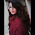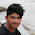## Monday, 12 November 2012

### Science & Technology Objective Test (Haryana Board)/NTSE Science Quiz-17

Science & Technology Objective Test
(Haryana Board)
NTSE Science Quiz-17

Q1: The speed of light in vacuum is

(a) 3 ×108 m/s
(b) 3 ×1010 m/s
(c) 3 ×105 m/s
(d) Zero

Q2: The angle of incidence for a ray of light is 45°. The angle of Reflection will be:

(a) 90°
(b) 45°
(c) 180°
(d) 0°

Q3: The focal length of a spherical mirror of radius of curvature 40 cm is

(a) 20 cm
(b) 40 cm
(c) 80 cm
(d) None of these

Q4: The mirror used in vehicles is

(a) Convex
(b) Concave
(c) Plane
(d) None of these

Q5: An object is placed at a distance of 10 cm in front of a plane mirror. The image is formed behind the mirror at a distance of

(a) 30 cm
(b) 10 cm
(c) 5 cm
(d) 40 cm

Q6: Which of the following is correct mirror formula

(a) f = v − u
(b) 1/f = 1/v - 1/u
(c) f = v + u
(d) 1/f = 1/v + 1/u

Q7: When ray of light goes from denser to rarer medium then it

(a) deviates towards the normal
(b) deviates away from the normal
(c) goes undeviated
(d) reflected back

Q8: The power of a lens of focal length 25 cm is

(a) + 40 D
(b) 0.4 D
(c) + 4 D
(d) – 2 D

Q9: The apparent depth of a coin inside water tab decreases due to

(a) Refraction of light
(b) Reflection of light
(c) Total internal reflection
(d) Dispersion of light

Q10: The S. I. unit of refractive index is

(a) meter
(b) cm
(c) watt
(d) no unit

Q11: The lens used in a simple microscope is

(a) Concave
(b) Convex
(c) Cylindrical
(d) None

Q12: What is the magnification of an astronomical telescope in normal adjustment, if the focal length of eye lens is 4 cm and focal length of object lens is 120 cm

(a) 30
(b) 60
(c) 120
(d) 124

Q13: The least distance of distinct vision for a normal human eye is

(a) 10 cm
(b) 25 cm
(c) 50 cm
(d) 60 cm

Q14: The image formed in a compound microscope is

(a) erect
(b) inverted
(c) sometimes erect, sometimes inverted
(d) none

Q15: What will be total magnification of a compound microscope, if the magnification of object lens is 5 and of eye lens is 10

(a) 2
(b) 15
(c) 50
(d) 10

Q16: Which of the following is not a primary colour

(a) Red
(b) Blue
(c) Green
(d) Yellow

Q17: Which colour of light is deviated least

(a) Red
(b) Blue
(c) Violet
(d) Green

Q18: The frequency of light of wavelength 5 ×10−7m in vacuum is

(a) 1.5×1014 Hz
(b) 6 ×1014 Hz
(c) 15 ×103 Hz
(d) None

Q19: Which of the following is a comet

(a) Halley
(b) Mars
(c) Venus
(d) Moon

Q20: Time period of a satellite in geo-stationary orbit is

(a) one year
(b) 24 hours
(c) 10 hours
(d) 12 hours

Q21: Parsec is the unit of

(a) Energy
(b) Work
(c) Distance
(d) Speed

Q22: The planet nearest to earth is

(a) Mercury
(b) Saturn
(c) Jupiter
(d) Venus

Q23: The first artificial satellite launched by India was

(a) Aryabhatta
(b) Rohini
(d) INSAT

Q24: Which of the following is the strongest electrolyte on the basis of its behaviour in the aqueous solution

(a) NH4Cl
(b) CH3COOH
(c) NaCl
(d) HCN

Q25: The equilibrium constant for the reaction N2 (g) + 3H2 (g) ≡ 2NH3 (g) is

(a) [N2][H2]3/[NH3]2

(b) [N2][H2]3/[NH3]

(c) 2[NH3]/[N2][H2]3

(d) [NH3]3/[N2][H2]3

Q26: Endothermic reactions are those in which

(a) Heat is evolved
(b) Heat is absorbed
(c) Temperature increases
(d) Light is produced

Q27: NaOH is a base because according to Arrhenius

(a) It has a bitter taste
(b) It can neutralize acid
(c) It turns red litmus paper blue
(d) It gives OH − ions in water

Q28: The pH of a solution is 4. When its pH changes to 2, then its H + ion concentration will

(a) decrease two times
(b) increase two times
(c) increase 100 times
(d) decrease 100 times

Q29: What is the percentage of Gold in 18-carat gold

(a) 75%
(b) 60%
(c) 67%
(d) 33%

Q30: The number of atoms in a molecule of sulphur are

(a) 4
(b) 8
(c) 2
(d) 6

Q31: Which metal is more reactive than hydrogen

(a) Cu
(b) Ag
(c) Fe
(d) Hg

Q32: AlCl3 reacts with aqueous NH3 to give

(a) Green precipitates
(b) White precipitates
(c) Red precipitates
(d) Blue precipitates

Q33: Sugar reacts with conc. H2SO4 to give

(a) CO2
(b) C
(c) CO
(d) SO2

Q34: Sodium hydroxide solution reacts with SO2 to form

(a) Na2SO3
(b) NaHSO3
(c) Na2SO4
(d) Na2S

Q35: Which of the following oxide is acidic

(a) CO
(b) N2O
(c) SiO2
(d) Fe2O3

Q36: Which of the following reaction cannot occur

(a) Zn (s) + CuSO4 (aq)→ ZnSO4 (aq) + Cu (s)
(b) 2AgNO3 (aq) + Cu (s)→ Cu(NO3)2 (aq) + 2Ag (s)
(c) Cu (s) + ZnSO4 (aq)→ CuSO4 (aq) + Zn (s)
(d) FeSO4 (aq) + Zn (s)→ ZnSO4 (aq) + Fe (s)

Q37: Which is not a favourable condition for the high yield of ammonia by Haber's process

(a) Excess of Hydrogen
(b) Excess of Nitrogen
(c) High Temperature
(d) High Pressure

Q38: The chemical formula of oleum is

(a) H2S2O7
(b) H2SO5
(c) H2SO3
(d) SO3

Q39: Haematite is an ore of

(a) Aluminium
(b) Iron
(c) Zinc
(d) Mercury

Q40: Brass is an alloy of copper and

(a) Ag
(b) Zn
(c) Al
(d) Fe

Q41: In the following reaction
SO2 (g) + 2H2S (g)→ 3S (s) + 2H2O (l)
SO2 is acting as an

(a) Acid
(b) Oxidising agent
(c) Reducing agent
(d) Base

Q42: Sulphide ore is separated from gangue by the process

(a) Froth floatation process
(b) Chemical method
(c) Electromagnetic separation
(d) Hydraulic washing

Q43: A metal which is purified by Liquation method has

(a) High melting point
(b) Low melting point
(c) Low density
(d) High density

Q44: The liquid metal is

(a) Bismuth
(b) Magnesium
(c) Mercury
(d) Sodium

Q45: Acid present in gastric juice is

(a) Hydrochloric Acid
(b) Citric Acid
(c) Sulphuric Acid
(d) Acetic Acid

Q46: Autotrophic mode of nutrition is found in

(a) Peepal
(b) Amarbel
(c) Human
(d) None of these

Q47: Gaseous exchange takes place in green leaves

(a) through Lenticels
(b) through Gills
(c) through Lungs
(d) through Stomata

Q48: Transportation of water and mineral salts takes place in

(a) Xylem Tissue
(b) Xylem Vessels
(c) Xylem Tracheids
(d) Phloem Tissue

Q49: The excretory unit in flatworms is

(a) Nephridia
(b) Osculum
(c) Nephron
(d) Flame cells

Q50: Green plants make food by

(a) Photo-oxidation
(b) Photorespiration
(c) Photosynthesis
(d) Photolysis

Q51: Name of respiratory organs in frog are

(a) Skin
(b) Lungs
(c) (A) & (B) both
(d) None of these

Q52: The pigment present in RBC is

(a) Erythrocin
(b) Haemoglobin
(c) Haematochrome
(d) None of these

Q53: The number of Nephrons present in one kidney in man are

(a) 100 Lacs
(b) 1 Lac
(c) 1000 Lacs
(d) 10 Lacs

Q54: Dark reaction of Photosynthesis was discovered by

(a) Melvin Calvin
(b) H. G. Khurana
(c) M. Calvin-Benson
(d) Landsteiner

Q55: Pick the odd one out

(a) Skin
(b) Gills
(c) Alveolus
(d) Lung

Q56: Lymph flows from

(a) Tissue to Heart
(b) Heart to Tissue
(c) Double circulation
(d) All of these

Q57: The shape of Bowman's capsule is like a

(a) Glass
(c) Spoon
(d) Cup

Q58: Where light reaction in chloroplast takes place

(a) Stroma
(c) Grana
(d) Double envelope

Q59: In case of severe respiratory problem the patient is kept on which instrument

(a) Ventilator
(b) Electrocardiograph
(d) Dialysis machine

Q60: Blood clotting is because of

(a) Fibrin
(b) Thrombin
(c) Thromboplastin
(d) All of above

Q61: The elimination of toxic nitrogenous waste and excess water in man is by

(a) Excretion
(b) Circulation
(c) Reproduction
(d) Pollution

Q62: Mode of nutrition in fungi is NOT

(a) Autotrophic
(b) Heterotrophic
(c) Saprophytic
(d) Parasitic

Q63: Tracheal system is found in

(a) Insects
(b) Human
(c) Fish
(d) Frog

Q64: How many dead cells are there in xylem tissue

(a) 4
(b) 3
(c) 2
(d) (b) & (c) both

Q65: Which blood cells are called 'Soldiers' of the body

(a) WBC
(b) Platelets
(c) RBC
(d) All of the above

(Answers will be posted soon...)

1: (a) 3 ×108 m/s
2: (b) 45°
3: (a) 20 cm [Focal length is half of the radius of curvature of spherical mirror]
4: (a) Convex [always give erect images, have wider back view]
5: (b) 10 cm
6: (d) 1/f = 1/v + 1/u
7: (b) deviates away from the normal
8: (c) + 4 D
9: (a) Refraction of light
10: (d) no unit
16: (d) Yellow
19: (a) Halley
11: (b) Convex
12:
13: (b) 25 cm
14: (b) inverted
15:
16: (d) Yellow
17: (a) red
18:
19: (a) Halley
20: (b) 24 hours
21: (c) Distance
22: (d) Venus
23: (a) Aryabhatta
24:
25:
26: (b) Heat is absorbed
27: (d) it gives OH- ions
28: (c) increases 100 times
29: (a) 75% (pure gold is 24-carat, calculate the ratio)
30: (b) 8
31: (c) Fe
32:
33:
34:
35:
36:
37:
38: (a) H2S2O7
39: (b) Iron
40: (b) Zn
41:
42: (a) Froth Floatation process
43: (a) High melting point
44: (c) Mercury
45: (a) Hydrochloric Acid
50: (c) Photosynthesis
51: (c) (A) & (B) both
55: (c) Alveolus (at anatomical level, rest are organs)
56: (a) Tissues to heart [*it travels from tissues to blood stream]
57: (d) Cup
58: (c) Grana
59: (a) Ventilator
60: (d) All of above
61: (a) Excretion
62: (a) Autotrophic
63: (a) Insects
64: ??
65: (a) WBC1.why the answers r missing????
plz give d answers completelyyy
waiting!!

2.Awesome but few ans are missing

3.This comment has been removed by the author.